U.S. Department of Transportation
1200 New Jersey Avenue, SE
Washington, DC 20590
202-366-4000

Federal Highway Administration Research and Technology
Coordinating, Developing, and Delivering Highway Transportation Innovations

 REPORT This report is an archived publication and may contain dated technical, contact, and link information
 Publication Number:  FHWA-HRT-12-031    Date:  August 2012
 Publication Number: FHWA-HRT-12-031 Date: August 2012

# User’s Guide: Estimation of Key PCC, Base, Subbase, and Pavement Engineering Properties From Routine Tests and Physical Characteristics

PDF files can be viewed with the Acrobat® Reader®

## CHAPTER 3. PCC MODELS

Prediction models were developed for PCC compressive strength, PCC flexural strength, PCC elastic modulus, PCC tensile strength, and CTE. The following limitations apply to all PCC models:

• A fundamental limitation for any model is that the relationship that exists between the predicted parameter and the regressors is only valid for the range of data that has been included in the dataset. Furthermore, the statistical modeling procedures for the most part assume that the variables are normally distributed within the dataset. For example, the relationships developed for PCC properties, (e.g., compressive strength prediction model) are applicable only for mixes with cement types 1 and 2. While one data point with type 3 cement exists in the database (a JRCP section) compared to 500 data sets with type 1 and type 2 cements, the strength gain pattern of a type 3 cement is clearly outcompeted by the other 2 cement types in the database. As a result, it might not be evident within this dataset that type 3 cements produce higher strengths, especially in the early ages.
• The model will reflect the intrinsic trends of the dataset used. For example, the data used for prediction of the 28-day compressive strength contains target low-strength and high-strength mix designs. If the primary means of achieving higher strengths for the States was to increase the cement content, the model will show a high correlation between the CMC and strength. However, there are multiple ways to enhance mix compressive strength, such as the use of lower w/c ratios, water-reducing agents, higher-strength aggregates, curing at higher temperatures and insulation, and use of type 3 cements. This is critical when the prediction models are implemented for estimating material properties.

## PCC Compressive Strength Models

Compressive strength is considered a fundamental strength parameter and is used at different stages of a project—design, QA, opening time, rehabilitation design, etc. The following models are offered for PCC compressive strength, each of which is discussed in subsections to follow:

• Compressive strength model 1: 28-day cylinder strength model (suitable for estimating design strength).
• Compressive strength model 2: Short-term cylinder strength model (suitable for estimating opening time).
• Compressive strength model 3: Short-term core strength model (suitable for in situ strength and opening time).
• Compressive strength model 4: All ages core strength model (suitable for estimating in situ strength at any age).
• Compressive strength model 5: Long-term core strength model (suitable for estimating long-term strength for rehabilitation design).

### Compressive Strength Model 1: 28-day Cylinder Strength Model

The 28-day compressive strength model developed for cylinder strength is as follows: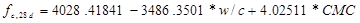Figure 3. Equation. Prediction model 1 for fc,28d.

Where:

f’c,28d = 28-day compressive strength, psi.
w/c = Water to cementitious materials ratio.
CMC = Cementitious materials content, lb/yd3.

The model statistics are shown in table 1. The model was developed using 42 data points, and the prediction has an R2 value of 54.44 percent and a root mean square error (RMSE) of 871 psi. Although it was compromised relative to the models discussed above, it provides a more meaningful model with a superior predictive ability. Table 2 provides details of the range of data used to develop the model.

Table 1. Regression statistics for selected prediction model for 28-day PCC cylinder strength.

 Variable Degrees of Freedom (DF) Estimate Standard Error t-Value Pr > |t| VIF Intercept 1 4,028.41841 1,681.71576 2.4 0.0215 0 w/c ratio 1 -3486.3501 2,152.99857 -1.62 0.1134 2.40903 CMC 1 4.02511 1.32664 3.03 0.0043 2.40903

Table 2. Range of data used for 28-day PCC cylinder strength.

 Parameter Minimum Maximum Average w/c ratio 0.27 0.71 0.42 Cementitious content 376 936 664 Compressive strength 3,034 7,611 5,239

Figure 4 and figure 5 show the predicted versus measured values and the residuals plot for the model, respectively. Figure 6 and figure 7 show the sensitivity of this model to w/c ratio and CMC. The change in compressive strength appears reasonable for both of the parameters for the range of values evaluated. They are also consistent with the data in the database. Within practical ranges, a change in CMC from 500 to 650 lb/ft3 increases the 28-day strength from approximately 4,700 to 5,300 psi for a w/c ratio of 0.4. Likewise, a decrease in w/c ratio from 0.5 to 0.35 increases the strength from 4,700 to 5,200 psi.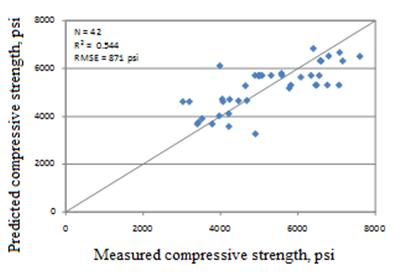Figure 4. Graph. Predicted versus measured for 28-day cylinder compressive strength model.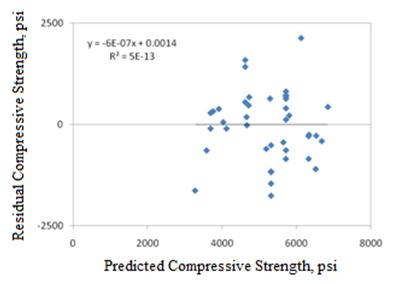Figure 5. Graph. Residual error plot for 28-day cylinder compressive strength model.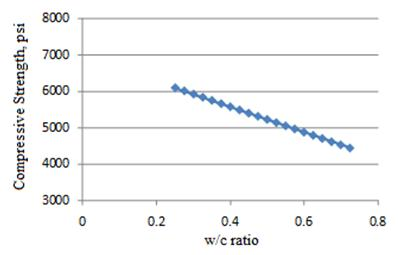Figure 6. Graph. 28-day compressive strength model sensitivity to w/c ratio.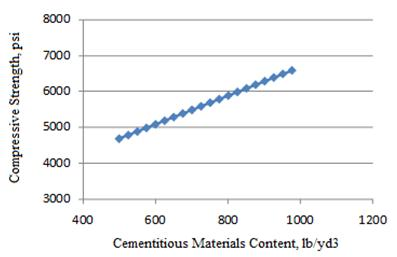Figure 7. Graph. 28-day compressive strength model sensitivity to CMC.

### Compressive Strength Model 2: Short-Term Cylinder Strength Model

The short-term cylinder compressive strength is expressed as follows: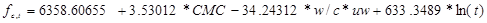Figure 8. Equation. Prediction model 2 for fc,t.

Where:

fc,t = Compressive strength at age t years, psi.
CMC = Cementitious materials content, lb/yd3.
w/c = Water to cementitious materials ratio.
uw = Unit weight, lb/ft3.
t = Short-term age up to 1 year.

The regression statistics for this model are presented in table 3, and details of the range of data used to develop the model are presented in table 4. The model was developed using 79 data points, and the prediction has an R2 value of 66.6 percent and an RMSE of 789 psi. The reason for an improved R2 compared to the 28-day strength model is not clear from these analyses.

Table 3. Regression statistics for short-term cylinder strength model.

 Variable DF Estimate Standard Error t-Value Pr > |t| VIF Intercept 1 6,358.60655 1,213.09762 5.24 < 0.0001 0 CMC 1 3.53012 0.90968 3.88 0.0002 2.15941 w/c × unit weight 1 -34.24312 11.00358 -3.11 0.0026 2.152 Ln(age) 1 633.3489 87.49625 7.24 < 0.0001 1.00604

Table 4. Range of data used for short-term cylinder strength model.

 Parameter Minimum Maximum Average w/c ratio 0.27 0.69 0.43 Cementitious content 376 936 660 Unit weight 124 151 143 Pavement age 0.0384 1.0000 0.3081 Compressive strength 2,480 10,032 5,256

Figure 9 and figure 10 show the predicted versus measured plot and the residual plot, respectively. Figure 11 through figure 13 show the sensitivity of this model to CMC, w/c ratio, and age, respectively. The trends are all reasonable. Figure 11 and figure 12 show the change in compressive strength at two ages, 28 days and 1 year, which are almost at the lower and upper bounds of ages included in this model. The plot in figure 13 can be considered a strength gain curve for typical unit weight and w/c ratios used in mix designs.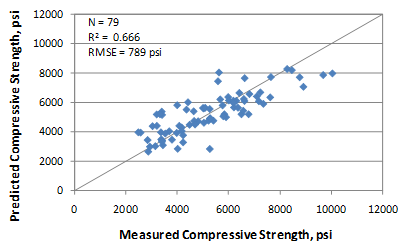Figure 9. Graph. Predicted versus measured for short-term cylinder compressive strength model.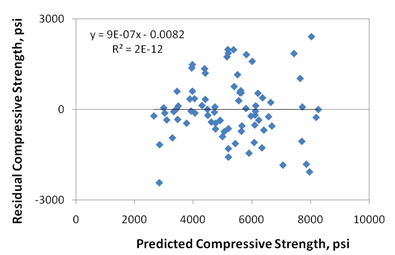Figure 10. Graph. Residual errors for short-term cylinder compressive strength model.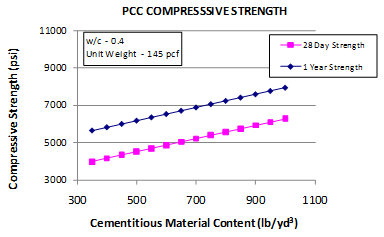Figure 11. Graph. Short-term cylinder compressive strength sensitivity to CMC.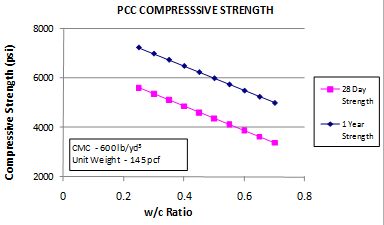Figure 12. Graph. Short-term cylinder compressive strength sensitivity to w/c ratio.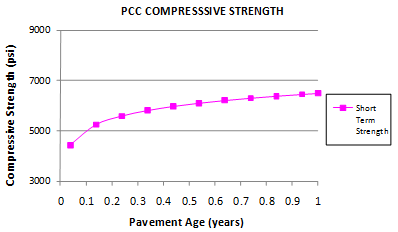Figure 13. Graph. Short-term cylinder compressive strength sensitivity to age.

### Compressive Strength Model 3: Short-Term Core Strength Model

The short-term core compressive strength model is as follows: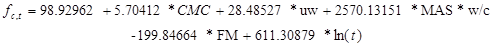Figure 14. Equation. Prediction model 3 for fc,t.

Where:

fc,t = Compressive strength at age t years, psi.
CMC = Cementitious materials content, lb/yd3.
uw = Unit weight, lb/ft3.
MAS = Maximum aggregate size, inch.
w/c = Water to cementitious materials ratio.
FM = Fineness modulus of fine aggregate.
t = Short-term age up to 1 year.

The regression statistics for this model are presented in table 5. The model was developed using 294 points, and the prediction has an R2 value of 67.61 percent and an RMSE of 1,122 psi. Table 6 provides details of the range of data used to develop the model.

Table 5. Regression statistics for short-term core strength model.

 Variable DF Estimate Standard Error t-Value Pr > |t| VIF Intercept 1 98.92962 1,544.34064 0.06 0.949 0 CMC 1 5.70412 0.36589 15.59 < 0.0001 1.23548 Unit weight 1 28.48527 10.59672 2.69 0.0076 1.0182 MAS × w/c ratio 1 2,570.13151 538.267 -4.77 < 0.0001 1.2201 FM 1 -199.84664 120.68288 -1.66 0.0988 1.01426 Ln(age) 1 611.30879 45.08962 13.56 < 0.0001 1.00026

Table 6. Range of data used for short-term core strength model.

 Parameter Minimum Maximum Average w/c ratio 0.27 0.69 0.42 Cementitious content 376 999 670 Unit weight 120 163 144 MAS 0.375 1.000 0.683 FM 2.50 4.37 3.05 Pavement age 0.0380 2.2160 0.4230 Compressive strength 1990 11,350 5,596

Figure 15 and figure 16 show the predicted versus measured plot and the residual plot, respectively. Figure 17 through figure 22 show the sensitivity of this model to CMC, unit weight, MAS, w/c ratio, FM, and age, respectively.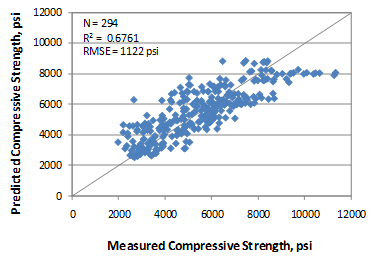Figure 15. Graph. Predicted versus measured for short-term core compressive strength model.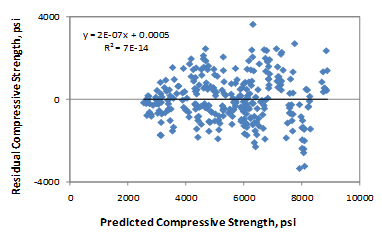Figure 16. Graph. Residual errors for short-term core compressive strength model.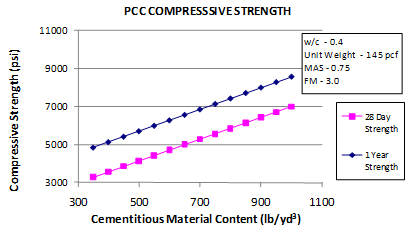Figure 17. Graph. Short-term core compressive strength sensitivity to CMC.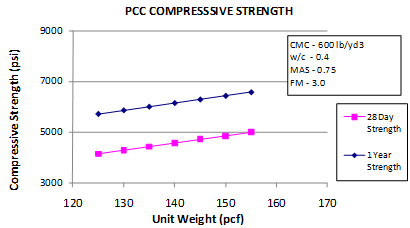Figure 18. Graph. Short-term core compressive strength sensitivity to unit weight.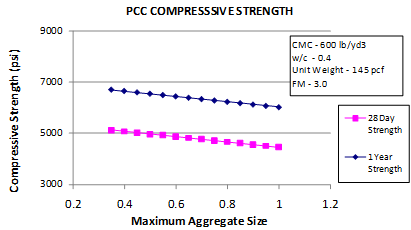Figure 19. Graph. Short-term core compressive strength sensitivity to MAS.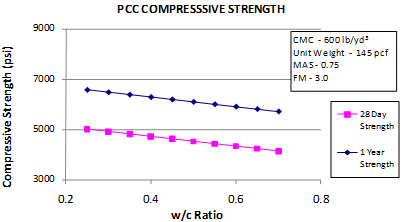Figure 20. Graph. Short-term core compressive strength sensitivity to w/c ratio.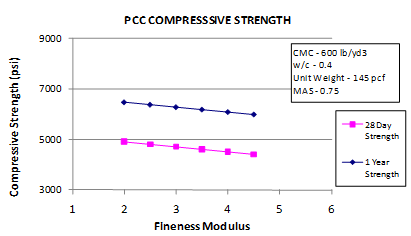Figure 21. Graph. Short-term core compressive strength sensitivity to fine aggregate FM.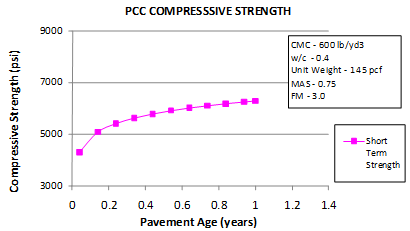Figure 22. Graph. Short-term core compressive strength sensitivity to age.

### Compressive Strength Model 4: All Ages Core Strength Model

The compressive strength for cores at all ages is estimated as follows: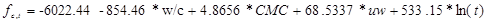Figure 23. Equation. Prediction model 4 for fc,t.

Where:

fc,t = Compressive strength at age t years, psi.
w/c = Water to cementitious materials ratio.
CMC = Cementitious materials content, lb/yd3.
uw = Unit weight, lb/ft3.
t = Short-term age in years.

The regression statistics for this model are presented in table 7. The model was developed using 580 data points, and the prediction has an R2 value of 55.38 percent and an RMSE of 992 psi. Table 8 provides details of the range of data used to develop the model.

Table 7. Regression statistics for all ages core strength model.

 Variable Estimate Standard Error t-Value Pr > |t| VIF Intercept -6,022.44 2,028.37 -2.97 0.0032 0 w/c ratio -854.46 675.86 -1.26 0.2069 2.15941 CMC 4.8656 0.5737 8.48 < 0.0001 2.152 Unit weight 68.5337 13.4368 5.1 < 0.0001 1.00604 Ln(age) 533.15 22.3343 23.87 < 0.0001 1.00026

Table 8. Range of data used for all ages core strength model.

 Parameter Minimum Maximum Average w/c ratio 0.00 0.72 0.43 Cementitious content 354 999 615 Unit weight 120 163 145 Pavement age 0.0380 45.3840 6.4320 Compressive strength 1,990 11,750 6,430

Figure 24 and figure 25 show the predicted versus measured plot and the residual plot, respectively.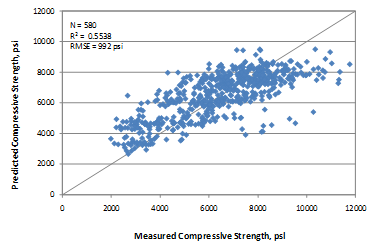Figure 24. Graph. Predicted versus measured for all ages core compressive strength model.Figure 25. Graph. Residual errors for all ages core compressive strength model.

Figure 26 through figure 29 show the sensitivity of this model to w/c ratio, CMC, unit weight, and age, respectively. Again, the sensitivity plots showing the variation in core compressive strength with changes in w/c ratio, CMC, and unit weight are presented for 28 days, 1 year, and 20 years. The rate of strength gain clearly is much higher in the short term (28 days to 1 year) than during the next 19 years. Figure 29 can be treated as the strength gain relationship representative of a typical mix (w/c of 0.4, CMC of 600 lb/yd3, and unit weight of 145 lb/ft3).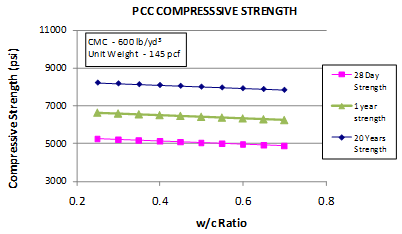Figure 26. Graph. All ages core compressive strength sensitivity to w/c ratio.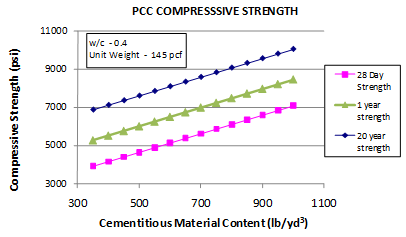Figure 27. Graph. All ages core compressive strength sensitivity to CMC.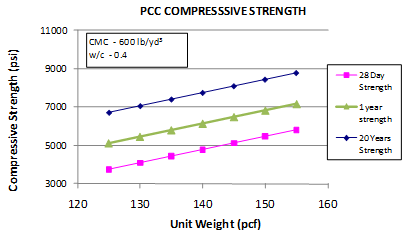Figure 28. Graph. All ages core compressive strength sensitivity to unit weight.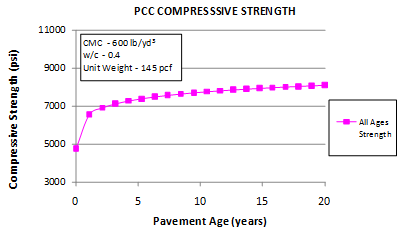Figure 29. Graph. All ages core compressive strength sensitivity to age.

### Compressive Strength Model 5: Long-Term Core Strength Model

The model developed for the long-term strength is expressed as follows: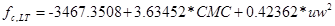Figure 30. Equation. Prediction model 5 for fc,LT.

Where:

fc,LT = Long-term compressive strength, psi.
CMC = Cementitious materials content, lb/yd3.
uw = Unit weight, lb/ft3.

The regression statistics for this model are presented in table 9. The model was developed using 201 data points, and the prediction has an R2 value of 18.03 percent and an RMSE of 1,179 psi. Table 10 provides details of the range of data used to develop the model.

Table 9. Regression statistics for long-term core strength model.

 Variable DF Estimate Standard Error t-Value Pr > |t| VIF Intercept 1 -3,467.3508 1,720.49637 -2.02 0.0452 0 Cementitious 1 3.63452 1.38354 2.63 0.0093 1.024 (Unit weight)2 1 0.42362 0.06634 6.39 < 0.0001 1.024

Table 10. Range of data used for long-term core strength model.

 Parameter Minimum Maximum Average Cementitious content 354 781 550 Unit weight 134 156 147 Compressive strength 4,315 11,750 7,655

Figure 31 and figure 32 show the predicted versus measured plot and the residual plot, respectively. This model does not have a good predictive ability (see figure 31). While there is no significant bias, the error in prediction is fairly high (see figure 32). This model needs to be used with caution. Additionally, other means to verify the value would be necessary, such as core tests.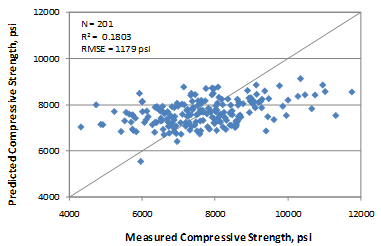Figure 31. Graph. Predicted versus measured for long-term core compressive strength model.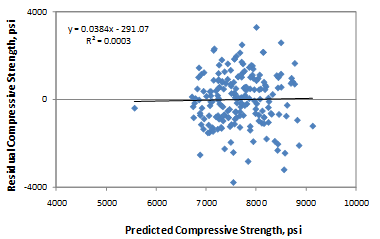Figure 32. Graph. Residual errors for long-term core compressive strength model.

### Relative Comparison of All Compressive Strength Models

The compressive strength models, like any other empirical model, reproduce the trends present in the datasets used for each correlation. It is highly recommended that a user estimate the strength based on as many models as possible with the information available at the time of analysis. This might provide a fair assessment of the ranges of compressive strength likely for the project and at different ages.

Figure 33 through figure 37 show the relationship between compressive strength and CMC, w/c ratio, and unit weight, respectively. Figure 36 and figure 37 show the strength gain at short- and long-term ages, respectively. Note that relationships have been plotted for typical values for all variables, and the raw data used in the models do not necessarily lie on the plots.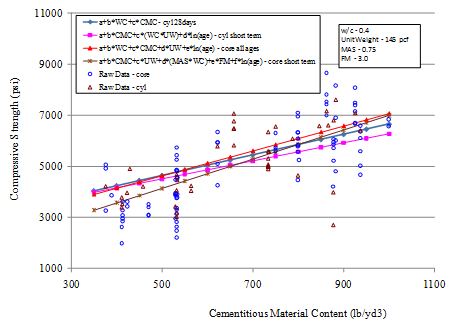Figure 33. Graph. Model compressive strength prediction for varying CMC.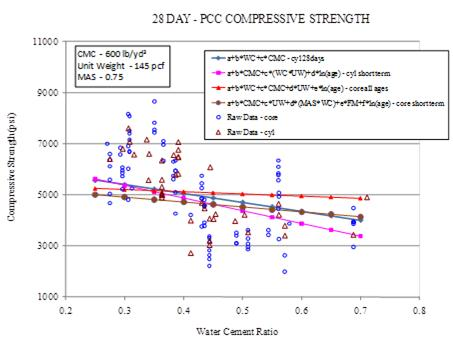Figure 34. Graph. Model compressive strength prediction for varying w/c ratio.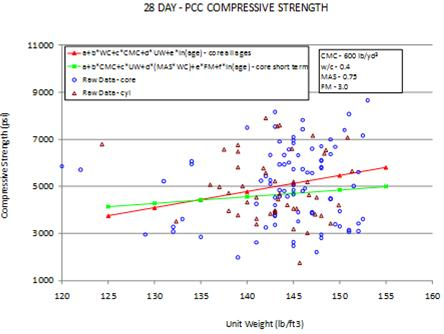Figure 35. Graph. Model compressive strength prediction for varying unit weights.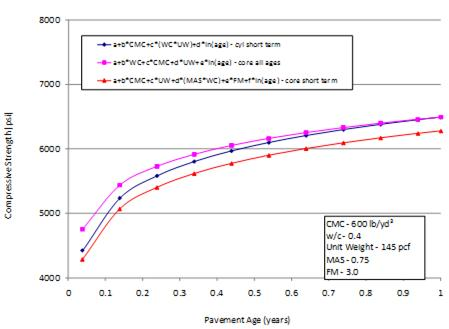Figure 36. Graph. Strength gain in the short-term predicted by three models.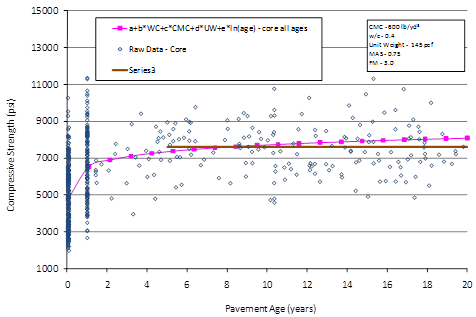Figure 37. Graph. Long-term strength gain predicted by the models.

The following observations can be made:

• The predictions are within 700 psi of each other for a given CMC (see figure 33). However, for more typical ranges of cement contents, the predictions are within 300 to 400 psi of each other. The short-term core compressive model has the steepest slope for this relationship.
• Figure 34 suggests that the predictions have a range as high as 1,500 psi for a given w/c ratio, especially at very high w/c ratios. However, within typical ranges (0.3 to 0.5), the models predict within a range of 250 to 800 psi. The range slightly increases at lower w/c ratios. The short-term cylinder strength has the highest slope in this case.
• Based on the trend presented in figure 35, for a given level of unit weight, the compressive strength predictions are within 200 to 300 psi for typical ranges of unit weight (140 to 145 lb/ft3). The prediction can vary by about 800 psi for very high unit weight values. Note that the short-term cylinder compressive strength model has not been included in this plot, as the variable appears as a transformed variable in the model and its effect cannot be isolated.
• Short-term strength predictions by all models that are relevant to short-term strengths show predictions within 200 to 400 psi of each other. The predictions are closer in value at as the age increases from 14 days to 1 year (see figure 36).
• Figure 37 suggests that the long-term strength predicted by the core all ages model is close to the strength predicted by the long-term model. This is essentially because the data used in this range are common to both models.

These observations illustrate the benefit of comparing predictions made by the various models available to obtain the range of strength that each project or observation could develop. Any other information to substantiate or validate the strength predictions should be utilized whenever possible, such as strength values from other projects that have used similar materials and mix design.

Federal Highway Administration | 1200 New Jersey Avenue, SE | Washington, DC 20590 | 202-366-4000
Turner-Fairbank Highway Research Center | 6300 Georgetown Pike | McLean, VA | 22101